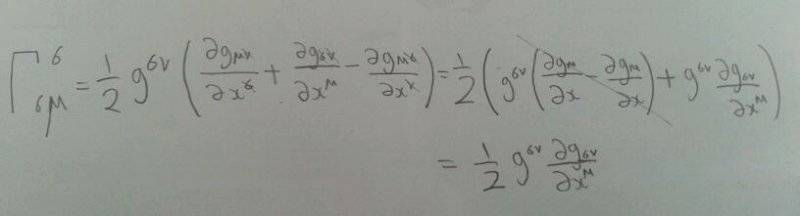# How to contract the christoffel symbol

TimeRip496
http://www.thephysicsforum.com/vlatex/pics/92_db3a451c7d105432675bef473582556e.png [Broken]

Anyone can help me? I am really stuck at here.

Last edited by a moderator:

http://www.thephysicsforum.com/vlatex/pics/92_db3a451c7d105432675bef473582556e.png [Broken]

Anyone can help me? I am really stuck at here.
Well, you should at least show your work so far, and exactly where you get "stuck". I.e., like in the homework forums.

Last edited by a moderator:
Gold Member
You might start with writing out how the Christoffel symbols are defined, and then contracting the two indices to see what you get.

TimeRip496
Well, you should at least show your work so far, and exactly where you get "stuck". I.e., like in the homework forums.
I get (0.5)(d/dxμ)(gσv)(gσv) . I don't even know to get that ln.

Sorry for my untidiness as I am still new to this.

Gold Member
If you don't show your intermediate steps, we can't really tell where you went wrong. By the way ##g_{\sigma\nu}g^{\sigma\nu}=\delta_\sigma^{~~\sigma}=n## where ##n## is the dimension of the manifold. So you definitely went wrong somewhere as your expression is the derivative of a constant so will equal 0.

TimeRip496
If you don't show your intermediate steps, we can't really tell where you went wrong. By the way ##g_{\sigma\nu}g^{\sigma\nu}=\delta_\sigma^{~~\sigma}=n## where ##n## is the dimension of the manifold. So you definitely went wrong somewhere as your expression is the derivative of a constant so will equal 0.I did that by cancelling out the indices.

Gold Member
Ok, but your final result ##\Gamma^\sigma_{\sigma\mu}=\frac{1}{2}g^{\sigma\nu}\partial_\mu g_{\sigma\nu}## is very different than the one you wrote in post #4.

You are pretty close to the answer. Perhaps the easiest way to move forward now is to expand the original statement ##\Gamma^\sigma_{\sigma\mu}=\partial_\mu (\ln\sqrt{g})## out to see what that looks like in terms of components ##g_{\mu\nu}## (it will be pretty hard to reverse-engineer this I think). The easiest way to work out the equation in the OP is to transform to a coordinate system in which the metric is diagonal (which can always be done), work out the derivatives in terms of components of the metric in that coordinate system, and then produce an equation which will work in any coordinate system. :)

TimeRip496
Ok, but your final result ##\Gamma^\sigma_{\sigma\mu}=\frac{1}{2}g^{\sigma\nu}\partial_\mu g_{\sigma\nu}## is very different than the one you wrote in post #4.

You are pretty close to the answer. Perhaps the easiest way to move forward now is to expand the original statement ##\Gamma^\sigma_{\sigma\mu}=\partial_\mu (\ln\sqrt{g})## out to see what that looks like in terms of components ##g_{\mu\nu}## (it will be pretty hard to reverse-engineer this I think). The easiest way to work out the equation in the OP is to transform to a coordinate system in which the metric is diagonal (which can always be done), work out the derivatives in terms of components of the metric in that coordinate system, and then produce an equation which will work in any coordinate system. :)
Thanks! I did finally manage to contract it. However, I have difficulty contracting Γσμμ. Is contracting it going to give us the same formula as shown above? I search online but I cant find any contraction of that christoffel symbol given above.